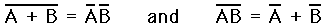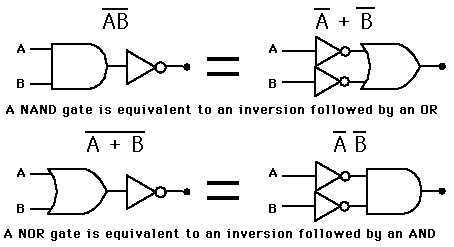# DeMorgan's TheoremShow in Gates

The most important logic theorem for digital electronics, this theorem says that any logical binary expression remains unchanged if we

1. Change all variables to their complements.
2. Change all AND operations to ORs.
3. Change all OR operations to ANDs.
4. Take the complement of the entire expression.

A practical operational way to look at DeMorgan's Theorem is that the inversion bar of an expression may be broken at any point and the operation at that point replaced by its opposite (i.e., AND replaced by OR or vice versa).

 Applications of DeMorgan's Theorem
Index

Electronics concepts

Digital Circuits

 HyperPhysics*****Electricity and magnetism R Nave
Go Back

# DeMorgan's Theorem in Gates

Two forms of DeMorgan's Theorem implemented with basic gates.Applications of DeMorgan's Theorem
Index

Electronics concepts

Digital Circuits

 HyperPhysics*****Electricity and magnetism R Nave
Go Back

# DeMorgan Applications

DeMorgan's Theorem is useful in the implementation of the basic gate operations with alternative gates, particularly with NAND and NOR gates which are readily available in IC form.

 NAND Gate Combinations NOR Gate Combinations XOR Gate with NANDs Negative Logic Gates
Index

Electronics concepts

Digital Circuits

 HyperPhysics*****Electricity and magnetism R Nave
Go Back